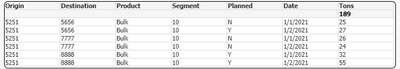# App Development

Announcements
Skip the ticket, Chat with Qlik Support instead for instant assistance.
cancel
Showing results for
Did you mean:Contributor II

## Select Most Recent Record

I am trying to select to most recent record where Planned = 'N' (for the given combination of dimensions).

Here is my table:I want the output to only show the following highlighted row:Is there a way to achieve this using a chart expression?

1 Solution

Accepted SolutionsMVP

Hi, you can using an expression like:

Sum({<Date={"\$(=Max({<Planned={N}>} Date))"},Planned={N}>} Tons)

Maybe you need the add a date format:

Sum({<Date={"\$(=Date(Max({<Planned={N}>} Date),'M/D/YYYY'))"},Planned={N}>} Tons)MVP

Hi, you can using an expression like:

Sum({<Date={"\$(=Max({<Planned={N}>} Date))"},Planned={N}>} Tons)

Maybe you need the add a date format:

Sum({<Date={"\$(=Date(Max({<Planned={N}>} Date),'M/D/YYYY'))"},Planned={N}>} Tons)Tags
Community Browser